## Cross-CapThe self-intersection of a one-sided Surface. It can be described as a circular Hole which, when entered, exits from its opposite point (from a topological viewpoint, both singular points on the cross-cap are equivalent). The cross-cap has a segment of double points which terminates at two Pinch Points'' known as Whitney Singularities.

The cross-cap can be generated using the general method for Nonorientable Surfaces using the polynomial function(1)

(Pinkall 1986). Transforming to Spherical Coordinates gives(2)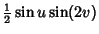(3)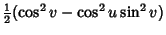(4)

for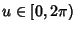and. To make the equations slightly simpler, all three equations are normally multiplied by a factor of 2 to clear the arbitrary scaling constant. Three views of the cross-cap generated using this equation are shown above. Note that the middle one looks suspiciously like Maeder's Owl Minimal Surface.

Another representation is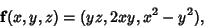(5)

(Gray 1993), giving parametric equations(6)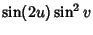(7)(8)

(Geometry Center) where, for aesthetic reasons, the- and-coordinates have been multiplied by 2 to produce a squashed, but topologically equivalent, surface. Nordstrand gives the implicit equation(9)

which can be solved forto yield(10)Taking the inversion of a cross-cap such that (0, 0,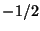) is sent togives a Cylindroid, shown above (Pinkall 1986).

The cross-cap is one of the three possible Surfaces obtained by sewing a Möbius Strip to the edge of a Disk. The other two are the Boy Surface and Roman Surface.

See also Boy Surface, Möbius Strip, Nonorientable Surface, Projective Plane, Roman Surface

References

Fischer, G. (Ed.). Plate 107 in Mathematische Modelle/Mathematical Models, Bildband/Photograph Volume. Braunschweig, Germany: Vieweg, p. 108, 1986.

Geometry Center. The Crosscap.'' http://www.geom.umn.edu/zoo/toptype/pplane/cap/.

Pinkall, U. Mathematical Models from the Collections of Universities and Museums (Ed. G. Fischer). Braunschweig, Germany: Vieweg, p. 64, 1986.